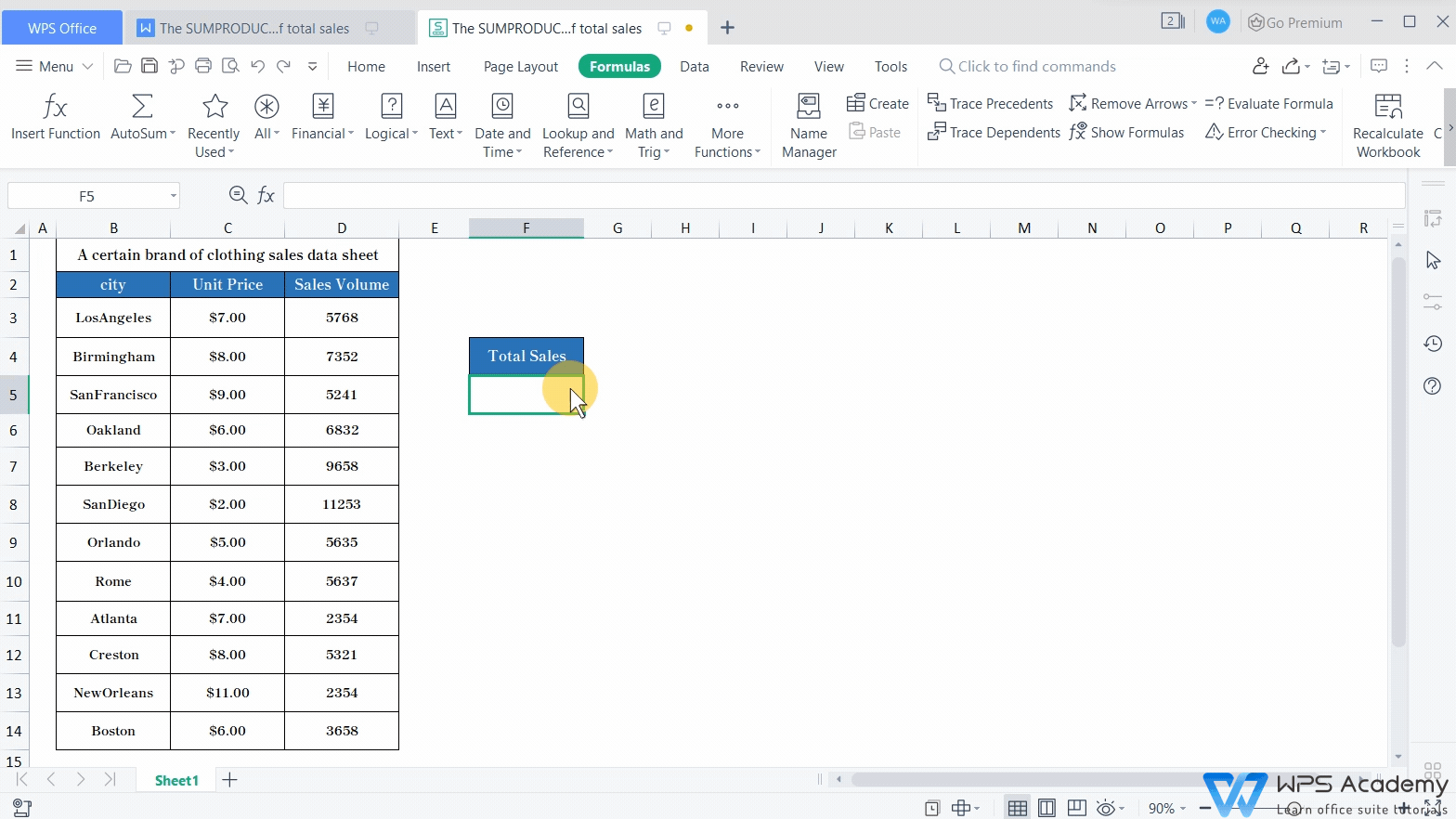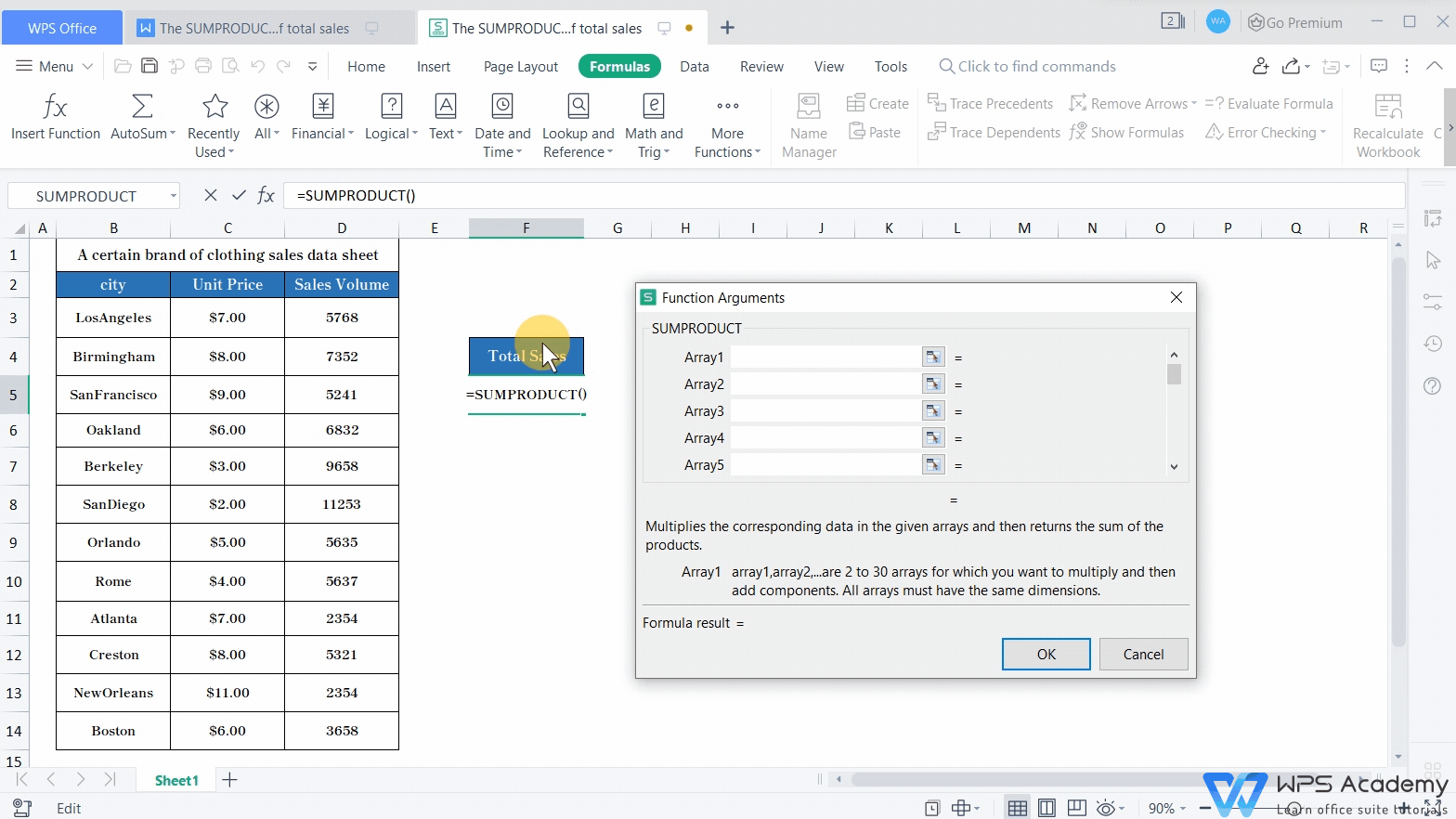# The SUMPRODUCT function for quick calculation of total sales

Uploaded time: August 27, 2021 Difficulty: Beginner# The SUMPRODUCT function for quick calculation of total sales

When using WPS Spreadsheets to process data, it is convenient to use the SUMPRODUCT function to multiply data and then sum the results that we get.

The spreadsheet for demonstration records sales amounts and prices of clothes in different cities. What can we do for a quick calculation of the total sales in all cities? The SUMPRODUCT function can realize it. This video could be also used in Microsoft Office Excel and Openoffice.

Place the cursor in cell F5. Then, click Insert Formula in the Formulas tab, and choose the SUMPRODUCT formula in the popup dialog.

The complete SUMPRODUCT function is =SUMPRODUCT(array1, [array2], ...).Then, there will be two arguments in the pop-up dialog. We can also add more arrays for statistics in terms of actual needs.

Here we enter C3:C14 in the array1input box and D3:D14 in the array2 box.

The basic logic is to multiply the two data in two different arrays and then sum the results getting from the previous calculation. In this example, run the SUMPRODUCT function in cell range C3:D14, then the calculation formula would be C3*D3+C4*D4...C14*D14.

Click OK to get the total sales of clothes for all cities.Now you can practice using the SUMPRODUCT function by yourself. Did you get it?